Chapter 7

As we know, the electric field energy density is: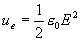(7.1)

Integrating energy density for the sphere of radius r, we can get the electric field energy: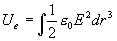(7.2)

With the electric field strength equation (4.2), then we can get: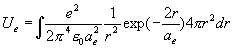(7.3)

Thus: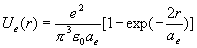(7.4)

Equation (7.4) is the electric energy distribution equation of electron. We find out that the electric field energy has the spherical exponential distribution inside of the electron.

When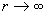, thus: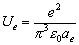(7.5)

Equation (7.5) is the electron’s electric field total energy equation.